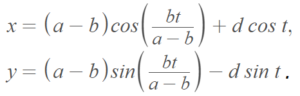# Hypocycloid Curve

A hypocycloid curve is a plane curve created by a point P on a small circle rolling around inside the circumference of a larger circle.

The parametric equations are## Cusps in the Hypocycloid Curve

Given that the larger circle’s diameter is a and the smaller circle’s diameter is b, we can define many unique hypocycloid curves. The ratio of a/b determines the number of cusps. For example, if the ratio is 3/1, the curve will have three cusps (i.e., it creates a deltoid curve).

If the ratio a/b is irrational, many more elaborate and interesting curves can be created, some of which resemble multi-petaled flowers or star shapes .Check out this Desmos page, where you can change the values for a and b to create a variety of different shapes.

Many authors restrict a and b to integers. For example, for a rotating ring gear type epicycloid reducer (Shin) or designing hypocycloid gear assembly for internal combustion engines . In engineering, the profile of some gear teeth is a combination of hypocycloid and epicycloid .

## History of the Hypocycloid Curve

Albrecht Durer (1471 to 1528) was the first to introduce the hypocycloid curve in his four-volume, 1,525 geometry tome The Art of Measurement with Compass and Straightedge . The curves were popularized in the latter half of the 20th Century with the invention of the Spirograph .

## References

Petaled hypocycloid curve created with Desmos.
Spirograph image: Kungfuman, CC BY-SA 3.0 , via Wikimedia Commons
 Chen, K. et al. (1999). Mathematical Explorations with MATLAB. Cambridge University Press.
 Parhusip, H. Arts revealed in calculus and its extension. International Journal of Statistics and Mathematics. Vol. 1(3), pp. 016-023, August, 2014.
 Bhattacharyya, B. (2013). Engineering Graphics. I.K. International Publishing House
 Simoson AJ (2008) Albrecht Dürer’s trochoidal woodcuts. Probl Resour Issues Math Undergraduate Stud (PRIMUS) 18(6):489–499
 Tsiotras, P. & Castro, L. Chapter 6 The Artistic Geometry of Consensus Protocols.

CITE THIS AS:
Stephanie Glen. "Hypocycloid Curve" From StatisticsHowTo.com: Elementary Statistics for the rest of us! https://www.statisticshowto.com/hypocycloid-curve/